Hostname: page-component-f7d5f74f5-cz72t Total loading time: 0 Render date: 2023-10-03T05:41:26.397Z Has data issue: false Feature Flags: { "corePageComponentGetUserInfoFromSharedSession": true, "coreDisableEcommerce": false, "coreDisableSocialShare": false, "coreDisableEcommerceForArticlePurchase": false, "coreDisableEcommerceForBookPurchase": false, "coreDisableEcommerceForElementPurchase": false, "coreUseNewShare": true, "useRatesEcommerce": true } hasContentIssue false

# Calculating conjugacy classes in Sylow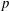$p$-subgroups of finite Chevalley groups of rank six and seven

Published online by Cambridge University Press:  01 April 2014

## Abstract

Core share and HTML view are not possible as this article does not have html content. However, as you have access to this content, a full PDF is available via the ‘Save PDF’ action button.

Let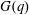$G(q)$ be a finite Chevalley group, where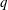$q$ is a power of a good prime$p$, and let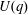$U(q)$ be a Sylow$p$-subgroup of$G(q)$. Then a generalized version of a conjecture of Higman asserts that the number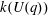$k(U(q))$ of conjugacy classes in$U(q)$ is given by a polynomial in$q$ with integer coefficients. In [S. M. Goodwin and G. Röhrle, J. Algebra 321 (2009) 3321–3334], the first and the third authors of the present paper developed an algorithm to calculate the values of$k(U(q))$. By implementing it into a computer program using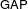$\mathsf{GAP}$, they were able to calculate$k(U(q))$ for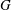$G$ of rank at most five, thereby proving that for these cases$k(U(q))$ is given by a polynomial in$q$. In this paper we present some refinements and improvements of the algorithm that allow us to calculate the values of$k(U(q))$ for finite Chevalley groups of rank six and seven, except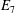$E_7$. We observe that$k(U(q))$ is a polynomial, so that the generalized Higman conjecture holds for these groups. Moreover, if we write$k(U(q))$ as a polynomial in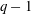$q-1$, then the coefficients are non-negative.

Under the assumption that$k(U(q))$ is a polynomial in$q-1$, we also give an explicit formula for the coefficients of$k(U(q))$ of degrees zero, one and two.

Type
Research Article
Information

## References

Alperin, J. L., ‘Unipotent conjugacy in general linear groups’, Comm. Algebra 34 (2006) 889891.CrossRefGoogle Scholar
André, C. A. M., ‘Basic characters of the unitriangular group (for arbitrary primes)’, Proc. Amer. Soc. 130 (2002) 19431954.CrossRefGoogle Scholar
Bosma, W., Cannon, J. and Playoust, C., ‘The Magma algebra system. I. The user language’, J. Symbolic Comput. 24 (1997) 235265.CrossRefGoogle Scholar
Bradley, J. D. and Goodwin, S. M., ‘Conjugacy classes in Sylow p-subgroups of finite Chevalley groups in bad characteristic’, Comm. Algebra (2013) to appear, arXiv:1201.1381.Google Scholar
Bürgstein, H. and Hesselink, W. H., ‘Algorithmic orbit classification for some Borel group actions’, Comp. Math. 61 (1987) 341.Google Scholar
Evseev, A., ‘Reduction for characters of finite algebra groups’, J. Algebra 325 (2011) 321351.CrossRefGoogle Scholar
The GAP group, ‘GAP—groups, algorithms, and programming, version 4.3’, 2002,http://www.gap-system.org.Google Scholar
Goodwin, S. M., ‘Relative Springer isomorphisms’, J. Algebra 290 (2005) 266281.CrossRefGoogle Scholar
Goodwin, S. M., ‘On the conjugacy classes in maximal unipotent subgroups of simple algebraic groups’, Transform. Groups 11 (2006) 5176.CrossRefGoogle Scholar
Goodwin, S. M., ‘Counting conjugacy classes in Sylow p-subgroups of Chevalley groups’, J. Pure Appl. Algebra 210 (2007) 201218.CrossRefGoogle Scholar
Goodwin, S. M. and Röhrle, G., ‘Calculating conjugacy classes in Sylow p-subgroups of finite Chevalley groups’, J. Algebra 321 (2009) 33213334.CrossRefGoogle Scholar
Goodwin, S. M. and Röhrle, G., ‘Rational points on generalized flag varieties and unipotent conjugacy in finite groups of Lie type’, Trans. Amer. Math. Soc. 361 (2009) 177206.CrossRefGoogle Scholar
Goodwin, S. M. and Röhrle, G., ‘Counting conjugacy classes in the unipotent radical of parabolic subgroups of${\rm GL}_n(q)$ ’, Pacific J. Math. 245 (2010) 4756.CrossRefGoogle Scholar
Greuel, G.-M., Pfister, G. and Schönemann, H., ‘Singular 3-1-1. A computer algebra system for polynomial computations’, Centre for Computer Algebra, University of Kaiserslautern, 2009, http://www. singular.uni-kl.de.Google Scholar
Higman, G., ‘Enumerating$p$ -groups. I. Inequalities’, Proc. Lond. Math. Soc. (3) 10 (1960) 2430.CrossRefGoogle Scholar
Himstedt, F. and Huang, S., ‘Character table of a Borel subgroup of the Ree groups$\mbox{}^2F_4(q^2)$ ’, LMS J. Comput. Math. 12 (2009) 153.CrossRefGoogle Scholar
Himstedt, F., Le, T. and Magaard, K., ‘Characters of the Sylow$p$ -subgroups of the Chevalley groups$D_4(p^n)$ ’, J. Algebra 332 (2011) 414427.CrossRefGoogle Scholar
Humphreys, J. E., Introduction to Lie algebras and representation theory , Graduate Texts in Mathematics 9 (Springer, New York, 1973).Google Scholar
Isaacs, I. M., ‘Counting characters of upper triangular groups’, J. Algebra 315 (2007) 698719.CrossRefGoogle Scholar
Le, T., ‘Counting irreducible representations of large degree of the upper triangular groups’, J. Algebra 324 (2010) 18031817.CrossRefGoogle Scholar
Lehrer, G. I., ‘Discrete series and the unipotent subgroup’, Compositio Math. 28 (1974) 919.Google Scholar
Robinson, G. R., ‘Counting conjugacy classes of unitriangular groups associated to finite-dimensional algebras’, J. Group Theory 1 (1998) 271274.CrossRefGoogle Scholar
Vera-López, A. and Arregi, J. M., ‘Conjugacy classes in unitriangular matrices’, Linear Algebra Appl. 370 (2003) 85124.CrossRefGoogle Scholar